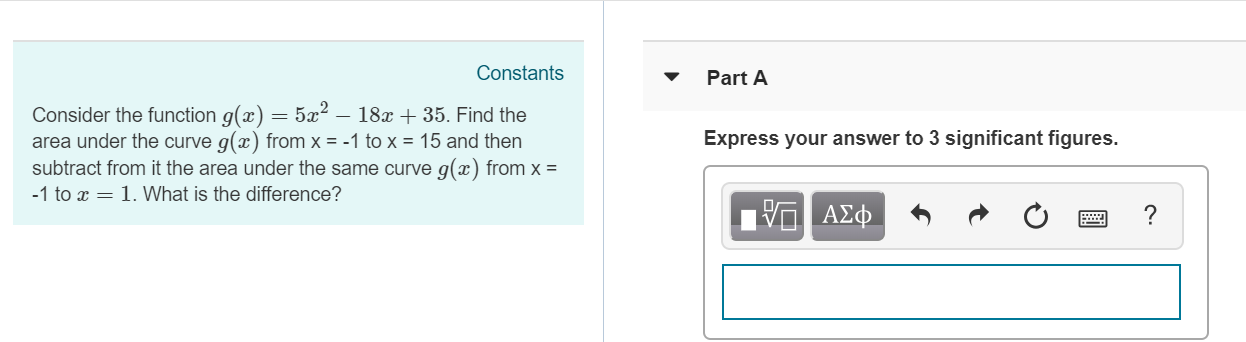# Constants Part A Consider the function g(z) 52 - 18a 35. Find the area under the curve g(x) from x =-1 to x = 15 and then subtract from it the area under the same curve g(z) from x 1 to r1. What is the difference? Express your answer to 3 significant figures.

Questionhelp_outlineImage TranscriptioncloseConstants Part A Consider the function g(z) 52 - 18a 35. Find the area under the curve g(x) from x =-1 to x = 15 and then subtract from it the area under the same curve g(z) from x 1 to r1. What is the difference? Express your answer to 3 significant figures. fullscreen

## Expert Answer

1 Rating

### Want to see this answer and more?

Experts are waiting 24/7 to provide step-by-step solutions in as fast as 30 minutes!*

*Response times may vary by subject and question complexity. Median response time is 34 minutes for paid subscribers and may be longer for promotional offers.
Tagged in
Math
Calculus

### Functions© 2021 bartleby. All Rights Reserved.